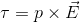# RBSE Solutions For Class 12 Physics Chapter 1: Electric Field | Textbook Important Questions & Answers

The solutions for all the questions on Chapter 1 Physics of RBSE Class 12 are provided here. Students can go through these questions to understand the concepts better and score well in the board examination. These solutions are solved by the team of experts to provide the best and accurate solutions to the questions. Visit BYJU’s RBSE Class 12 solutions page to get the answers for textbook questions of all subjects.

## Multiple Choice Questions

Q1: Two identical charges separated by a distance of 3m experience a force of repulsion of 16N, the magnitude of each charge is

1. 2μC
2. 40μC
3. 4μC
4. 80μC

Q2: The force acting between the two charges is 8N. If the separation between them is tripled then the force acting between them will be

1. F
2. F/3
3. F/9
4. F/27

Q3: Two-point charges +9e and +e are at a separation of 16m. Where on the line joining them another charge q must be put so it remains in equilibrium?

1. 24 cm away from +9c
2. 12 cm away from +9c
3. 24 cm away from +e
4. 12 cm away from +e

Q4: Two identical spheres having unequal and opposite charges are 90 cm apart. They are now made constant and then again separated by the same distance. Now they repel each other by a force of 0.025 N. Find the charge on each sphere.

1. 1.5 μC
2. 1.5 C
3. 3 C
4. 3 μC

Q5: On putting a glass plate in between the two charges the electrostatic force between them as compared to earlier is

1. more
2. less
3. zero
4. Infinite

Q6: Four equal and like charges are placed on the four vertices of a square. If the field intensity due to any of the charge at the centre is E then the net electric field intensity at the centre of the square will be

1. Zero
2. E
3. E/4
4. 4E

Q7: On placing a dipole in a uniform electric field it is acted upon by a

1. Torque only
2. Force only
3. Both force and torque
4. Neither for nor torque

Q8: For the same distance from the centre of dipole the ratio of electric fields at longitudinal and transverse position is

1. 1:2
2. 2:1
3. 1:4
4. 4:1

Q9: The force of attraction between +5 μC and -5 μC charges kept at some distance apart is 9N. When the two charges are made to contact and then separated again by the same distance the force acting between them becomes

1. Infinite
2. 9 x 109 N
3. 1N
4. zero

Q10: Two equal but unlike charged objects are kept at some distance apart with a force F acting between them. If 75% charge of one of them is somehow transferred to the other then the new force between them is

1. F/16
2. 7F/16
3. 9F/16
4. !5F/16

## Very Short Answer Type Questions

Q1: Write the value of one quantum of charge.

Answer: The value of one quantum of charge is 1.602176634×10−1

Q2: The electrostatic force between two protons separated by a distance r is F. If the protons are replaced by electrons then what will be the force?

Answer: The force between the two electrons will also be F

Q3: The force exerted by one charge on another is F. In presence of a third charge what will be the force on the second charge by the first charge.

Answer: The force on the second charge by the first charge will be F even when a third charge is present

Q4: Name the experiment with which the quantum nature of charge was established?

Answer: The experiment is Milliken’s oil drop experiment

Q5: What is the force acting on a charge placed in an electric field E?

Answer: The force acting on the charge q is given by, F= qE

Q6: What is the effect of speed of a charged particle on its charge and mass?

Answer: If the speed is of the order of the speed of light then the mass increases as the speed of light increases. However, the charge is unaffected by the speed of light.

Q7: What is the magnitude of the intensity of the electric field that can balance the weight of an electron? Given: e = 1.6 × 10−19 C, m = 9.1× 1031 Kg

The magnitude of the electric field is given by

eE = mg

E = mg/e

E = 9.1× 1031 ×9.8/1.6 × 10−1

E= 5.57 × 10−11 N/C

Q8: Give the definition of electric dipole moment.

Answer: Electric Dipole moment is given by the product of magnitudes of the charges and the distance between them. It is given by the equation

p = q.2a

where,

p = magnitude of the charge

2a = distance between the charges

Q9: Write the condition for an ideal electric dipole

Answer: An ideal dipole is the combination of two opposite charges of the same magnitude having a small distance between them.

Q10: What is the net force on an electric dipole in a uniform electric field?

Answer: Dipole meaning two opposite charges. The force (F) of the positive charge +q is +qE and the force on the negative charge -q is -qE. Therefore the net force on an electric dipole in a uniform electric field is zero.

Q1: What is meant by frictional electricity? Describe its origin.

Answer: Frictional electricity is produced when two objects are rubbed together. When the objects are rubbed together there is a transfer of electrons from one object to another. The object which loses the electron gets positively charged and the object receiving the electron will be negatively charged. Let us take the example of a glass rod and silk cloth. When they are rubbed together, the glass rod gets negatively charged and silk cloth gets equally positively charged.

Q2: State Coulomb’s law for the electric force between two point charges at rest

Answer: Coulomb’s law also known as the Coulomb’s inverse square law states that two like charges repel each other or two opposite charges attract each other with a force proportional to the product of the two charges and inversely proportional to the square of the distance between them.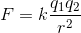q1,q2 = charges

r =distance between the charges

Q3: Write the law of superposition of forces

Answer: According to the law of superposition of force the net force acting on a charge is equal to the sum of the individual forces.

Q4: What is meant by electric field lines? Write its two properties

Answer: The electric field lines are imaginary lines drawn in a region where the electric field exists. The tangent drawn to the field lines at any point shows the direction of the electric field vector. For an isolated positive charge the field lines are radially directed outward. For an isolated negative charge, the direction of field lines is radially inward.

Q5: Explain the law of conservation of charge.

Answer: According to the law of conservation of charge, the total charge in a closed system remains constant. The amount of positive charge minus the amount of negative charge in the universe is always conserved.

Q6: Define the relative permittivity of a medium.

Answer: The relative permittivity of the medium is the ratio of the electrostatic force between two charges kept in vacuum to the force between the same two charges kept at the same distance in the particular medium.

Q7: How can a metallic sphere be charged without touching it?

Answer: The induction charging is a charging process in which the object is charged without actually being touched. The charging by induction process is where the charged object is held near an uncharged sphere that is grounded on a neutrally charged material. The charge flows between two objects and the uncharged sphere develops a charge with opposite polarity.

Q8: What is q1+q2 =0 in reference to charges?

Answer: If q1+q2 is equal to zero it means that the total force acting on the system is also zero.

Q9: Explain quantisation of charge.

Answer: The quantisation of electric charge means that the total charge of the system is an integral multiple of individual charge (e).

q= ne

Where

n = 0, ±1, ±2, ±3, …..

e = charge of an electron

Q10: A dipole is kept in a uniform electric field. Show that it will not have a translatory motion

Answer: An electric dipole has two equal and opposite charges. These charges are separated by a finite distance. When they are kept in a uniform external field they will experience two equal and opposite forces and the net force acting on the dipole is zero. Therefore, the dipole will not have a translatory motion.

Q11: Explain why the test charge employed should be infinitesimally small for determining the electric field due to a point charge.

Answer: The basic reason is that the test charge should not disturb the electric field. When the test charge is infinitesimally small then it will not alter the field being measured.

Q12: A copper sphere of 2 gram contains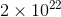atoms. The nucleus of each atom has a charge 29e. What fraction of electrons should be removed from the sphere to give it a charge of 2μC?

Given:

Total number of atoms =The charge on the nucleus of each atom = 29e

q = 2μC =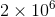C

Solution:

Total charge ne = q

n = q/e

= 2× 106/1.6 × 10−19

=1.25× 1013

Total number of electrons in the sphere = 29 × 2× 1022

Fraction of electron removed = 1.25× 1013/29 × 2× 1022

Fraction of electron removed = 2.16 × 10-11

Q13: Consider two identical metallic spheres of exactly the same mass. One of them is given some negative charge and the other is charged positively by the same amount, will there be any difference in the masses of the sphere after charging? If yes, Why?

The mass of the metallic sphere charged negatively increases. The electrons are injected into the sphere in order to give the sphere a negative charge. Therefore, the mass of the sphere increases.

The mass of the metallic sphere charged positively decreases. The electrons are removed from the metallic sphere to give the sphere a positive charge, this results in reduced mass.

Q14: On moving away from a point charge the electric field due to charge decreases. The same is true for an electric dipole. Does the electric field for both these cases decrease at the same rate?

Answer: The rate of decrease in the electric field is different for point charge and electric dipole. The electric field decreases by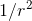for a point charge but for an electric dipole it decreases by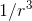. Therefore the electric field intensity reduces more rapidly for a dipole along the axial line than for a single charge.

## Essay Type Questions

Q1: Define Coulomb’s law for the electrostatic force between two charges, and discuss its limitations. Using this law define 1 coulomb of charge.

Definition of Coulomb’s Law

Coulomb’s Law or Coulomb’s Inverse Square Law states that the magnetic of the electric force of attraction or repulsion acting between two point charges at rest is directly proportional to the product of the charges and inversely proportional to the square of the distance between them. This force acts along the line joining the two charges and it also depends on the nature of the medium between the charges.

If F is the force between two charges then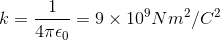Where

q1,q2 = two charges separated at a r

r = distance between the two charges

k = proportionality constant

ε0 = permittivity of free space

Limitations of Coulombs Law

• Coulomb’s Law holds good only for point charges at rest
• Coulomb’s Law cannot be applied where the charges are in an arbitrary shape. If the charges are in arbitrary shape the distance between the charges cannot be determined.
• The charge cannot be used to determine the charge on the bigger planets
• Coulomb’s Law can be only applied in those cases where the inverse square law is obeyed.

Definition of 1 Coulomb of charge from Coulomb’s Law

If the force acting between the two equal charges kept at a distance of 1m is 9×109 N then the magnitude of each charge is equal to 1 Coulomb.

Q2: Give the definition of an electric field. Derive the expression for the electric field due to a point charge. If another charge q1 is brought in this field what will be the electric force acting on it?

Definition of Electric Field: The space around a charge or a system of charges in which other charged particles experience electrical force is called an electrostatic field or electric field. Any charged particle kept in this field will experience a force called electrostatic force.

Expression for electric field due to a point charge

We will consider a charge +q kept in free space at the point O. Let us consider a point P at a distance r from the point charge. In order to calculate the electric intensity at point P we should keep a test charge +q1 at P.

According to Coulomb’s Law, the electrostatic force acting on the charge +q1 is given by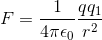The direction of the electric force is from O to P

Let us divide both sides of the equation by q1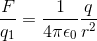But F/q1 is the force per unit positive test charge at P.The force per unit charge is also called as electric intensity.

Therefore, the electric intensity at P is given by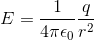If another charge q1 is brought in this field the electric force acting on it is given by Coulomb’s force.

Q3: What is meant by an electric dipole. Define electric dipole moment. Derive an expression for intensity of the electric field at an axial point of an electric dipole

Electric dipole: Two equal and opposite charges separated by a small distance is called Electric dipole.

Electric dipole moment: The product of either one of the charges and the distance between the two charges is called an electric dipole moment. The electric dipole moment is given by the letter p.

p = q(2a)

Where q is a charge and 2a is the distance between two charges

Expression for the intensity of the electric field at an axial point of an electric dipole

Consider two charges +q and -q kept at the points B and A. The moment of the dipole is given by p = q(2a). Where q is the magnitude of either one of the charges and 2a is the distance between the two charges. Consider a point M on the axial line at a distance r from the centre O of the dipole.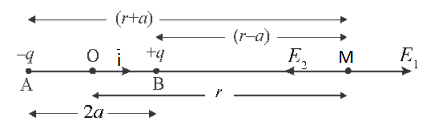Electric field at M due to the charge +q at B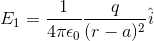(in the direction B to M)

The electric field at M due to the charge -q at A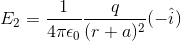(in the direction M to A)

Here i is the unit vector. The net electric field at point M is equal to the sum of E1 and E2.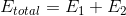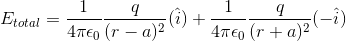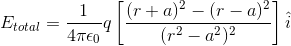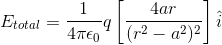Since dipole moment p =q(2a), the above equation can be rewritten as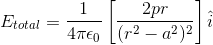If the value of a<< r then the value of “a” can be considered negligible.

Therefore, the value of the net electric field can be written as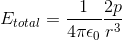The magnitude of the electric field is inversely proportional to the cube root of r. Therefore, the electric field intensity reduces more rapidly for a dipole along the axial line than for a single charge.

Q4: Derive the expression for the torque acting on a dipole placed in a uniform electric field.

Answer: Torque is the force that causes a dipole to rotate about an axis. It is a vector quantity and its direction depends on the direction of the force applied. Let us consider the charges +q and -q kept at a distance d. The dipole is kept in a uniform electric field of strength E and the dipole makes an angle θ with the electric field.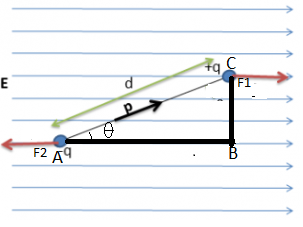The force on the charge +q due to the electric field is given by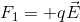The force on the charge -q due to the electric field is given by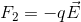The torque on the dipole is given by

Torque = Force on a single charge X perpendicular distance between the line of action of the forces. From the figure, we know the perpendicular distance is BC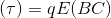From the figure, we know

sinθ =BC/d

BC = dsinθ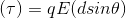The dipole moment p =qd, where q is the charge and d is the distance between the charges. Therefore the equation for torque can be written as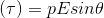The vector form of the equation of torque is given by